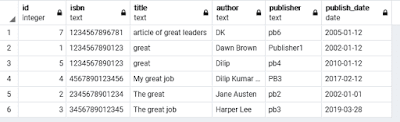### Arithmetic aggregation operators in MongoDB

\$abs: \$abs returns absolute value of the number.

\$add: \$add use to summation numbers and date it returns same as added number and new added date. If you are adding date mean first expression is date and second then second expression (number) treats as millisecond. \$add accept any number of expression.

\$ceil: \$ceil returns the smallest integer greater than or equal to the specified number.

\$divide: \$divide returns the result of dividing the first number by the second. \$divide accepts two argument expressions.

\$exp: \$exp Euler’s number (i.e. e ) to the specified exponent and returns the result.

\$floor: \$floor returns the largest integer less than or equal to the specified number.

\$ln: \$ln calculates the natural log of a number.

\$log: \$log calculates the log of a number in the specified base.

\$log10:\$log10 calculates the log base 10 of a number.

\$mod:\$mod returns the remainder of the first number divided by the second. \$mod accepts two argument expressions.

\$multiply: \$multiply multiplies numbers to return the product. \$multiply  accepts any number of argument expressions.

\$pow: \$pow raises a number to the specified exponent.

\$sqrt: \$sqrt calculates the square root.

\$subtract: \$subtract returns the result of subtracting the second value from the first. If the two values are numbers, return the difference. If the two values are dates, return the difference in milliseconds. If the two values are a date and a number in milliseconds, return the resulting date. \$subtract accepts two argument expressions. If the two values are a date and a number, specify the date argument first as it is not meaningful to subtract a date from a number.

\$trunc:\$trunc truncates a number to its integer.